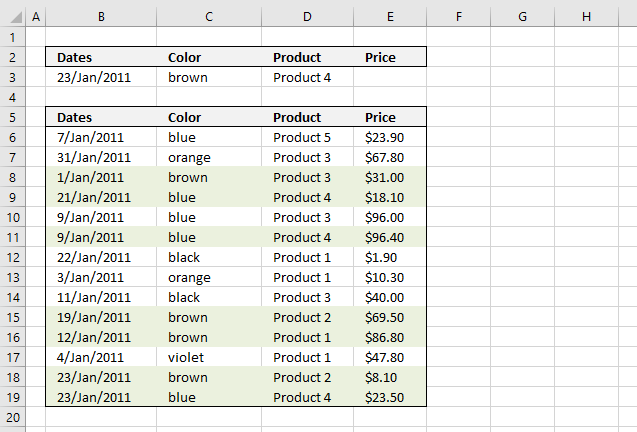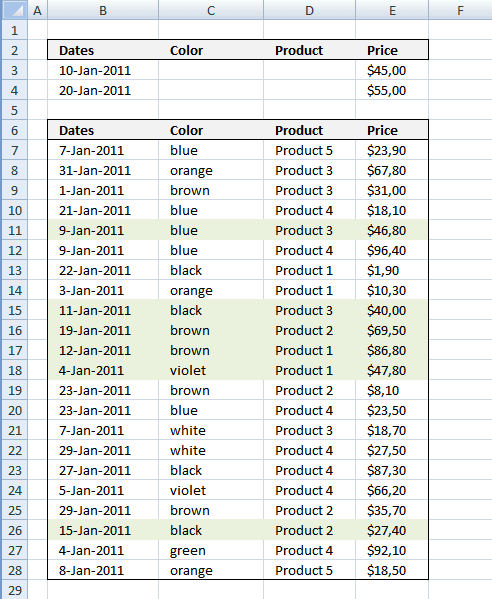Author: Oscar Cronquist Article last updated on January 14, 2019The image above shows you how to highlight rows with multiple criteria using OR logic. The criteria are found in row 3.

A row is highlighted if:

• Date criterion is found in column B or
• Color criterion is found in column C or
• Product criterion is found in column D or
• Price criterion is found in column E

Conditional formatting formula:

=SUMPRODUCT((\$B\$3=\$B6)+(\$C\$3=\$C6)+(\$D\$3=\$D6)+(\$E\$3=\$E6))

Explaining formula in cell D11

Step 1 - Understand how relative and absolute cell references work

In cell B6 the formula is: =SUMPRODUCT((\$B\$3=\$B6)+(\$C\$3=\$C6)+(\$D\$3=\$D6)+(\$E\$3=\$E6))

and in cell D11 the formula has changed to =SUMPRODUCT((\$B\$3=\$B11)+(\$C\$3=\$C11)+(\$D\$3=\$D11)+(\$E\$3=\$E11))

Step 2 - OR logic is created using + signs between criteria

=SUMPRODUCT((\$B\$3=\$B11)+(\$C\$3=\$C11)+(\$D\$3=\$D11)+(\$E\$3=\$E11))

becomes

=SUMPRODUCT((40566=40552)+("brown"="blue")+("Product 4"="Product 4")+(""=96,40))

becomes

=SUMPRODUCT(FALSE+FALSE+TRUE+FALSE)

becomes

=SUMPRODUCT(0+0+1+0) and returns 1. (TRUE)

Cell D11 is highlighted

How to apply conditional formatting formula

1. Select cells B6:E27
2. Press with left mouse button on "Home" tab
3. Press with left mouse button on "Conditional Formatting" button
4. Press with left mouse button on "New Rule.."
5. Press with left mouse button on "Use a formula to determine which cells to format"
6. Type =SUMPRODUCT((\$B\$3=\$B6)+(\$C\$3=\$C6)+(\$D\$3=\$D6)+(\$E\$3=\$E6)) in "Format values where this formula is TRUE" window.
7. Press with left mouse button on "Format.." button
8. Press with left mouse button on "Fill" tab
9. Select a color for highlighted cells.
10. Press with left mouse button on "Ok"
11. Press with left mouse button on "Ok"
12. Press with left mouse button on "Ok"
Note: Conditional formatting is volatile meaning when the worksheet is recalculated all conditionally formatted cells are recalculated, this may slow down your worksheet considerably.

Date range and price range criteria

Let's set up a new sheet with a date range as a criterion and a price range as another criterion.

A row is highlighted if:

• A date is smaller or equal to cell B4 and the same date is larger or equal to cell B3 or
• Color criterion is found in column C or
• Product criterion is found in column D or
• A price is smaller or equal to cell E4 and the same price is larger or equal to cell E3Conditional formatting formula:

=SUMPRODUCT((\$B\$3<=\$B7)*(\$B\$4>=\$B7)+(\$C\$3=\$C7)+(\$D\$3=\$D7)+(\$E\$3<=\$E7)*(\$E\$4>=\$E7))

How to apply conditional formatting formula

1. Select cells B6:E27
2. Press with left mouse button on "Home" tab
3. Press with left mouse button on "Conditional Formatting" button
4. Press with left mouse button on "New Rule.."
5. Press with left mouse button on "Use a formula to determine which cells to format"
6. Type =SUMPRODUCT((\$B\$3<=\$B7)*(\$B\$4>=\$B7)+(\$C\$3=\$C7)+(\$D\$3=\$D7)+(\$E\$3<=\$E7)*(\$E\$4>=\$E7)) in "Format values where this formula is TRUE" window.
7. Press with left mouse button on "Format.." button
8. Press with left mouse button on "Fill" tab
9. Select a color for highlighted cells.
10. Press with left mouse button on "Ok"
11. Press with left mouse button on "Ok"
12. Press with left mouse button on "Ok"

Get the Excel filequickly-highlight-records-in-a-list-using-multiple-criteria.xlsx